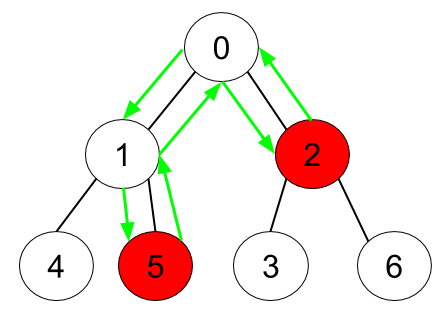1443. Minimum Time to Collect All Apples in a Tree
Medium
3.3K
275

Given an undirected tree consisting of `n` vertices numbered from `0` to `n-1`, which has some apples in their vertices. You spend 1 second to walk over one edge of the tree. Return the minimum time in seconds you have to spend to collect all apples in the tree, starting at vertex 0 and coming back to this vertex.

The edges of the undirected tree are given in the array `edges`, where `edges[i] = [ai, bi]` means that exists an edge connecting the vertices `ai` and `bi`. Additionally, there is a boolean array `hasApple`, where `hasApple[i] = true` means that vertex `i` has an apple; otherwise, it does not have any apple.

Example 1:```Input: n = 7, edges = [[0,1],[0,2],[1,4],[1,5],[2,3],[2,6]], hasApple = [false,false,true,false,true,true,false]
Output: 8
Explanation: The figure above represents the given tree where red vertices have an apple. One optimal path to collect all apples is shown by the green arrows.
```

Example 2:```Input: n = 7, edges = [[0,1],[0,2],[1,4],[1,5],[2,3],[2,6]], hasApple = [false,false,true,false,false,true,false]
Output: 6
Explanation: The figure above represents the given tree where red vertices have an apple. One optimal path to collect all apples is shown by the green arrows.
```

Example 3:

```Input: n = 7, edges = [[0,1],[0,2],[1,4],[1,5],[2,3],[2,6]], hasApple = [false,false,false,false,false,false,false]
Output: 0
```

Constraints:

• `1 <= n <= 105`
• `edges.length == n - 1`
• `edges[i].length == 2`
• `0 <= ai < bi <= n - 1`
• `hasApple.length == n`
Accepted
98.6K
Submissions
157.1K
Acceptance Rate
62.7%

Seen this question in a real interview before?
1/4
Yes
No

Discussion (0)

Related Topics